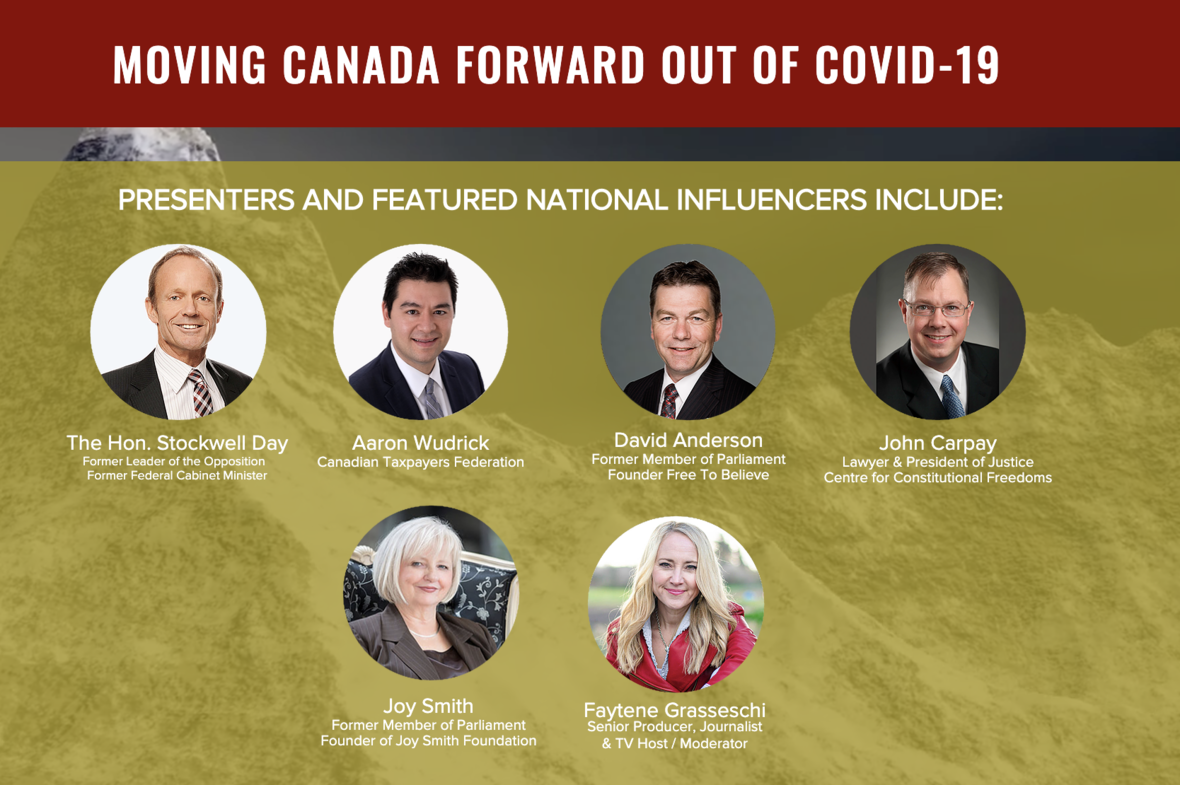Like   Tweet   Pin   +1   in
 /* styles */ May 15, 2020

## THIS IS IT!

Today is the last day that you can purchase a membership for the Conservative Party of Canada (CPC) to vote in their leadership race this summer. Because of the nature of politics in Canada, many analysts agree that whoever wins this race could very well be the next Prime Minister.

This is not an endorsement of a party. This is an encouragement to engage at a critical point of our democratic journey as a nation.

Issues such as parent rights, our relationship with Israel, gendercide, how we care for the elderly, the federal debt, taxes and constitutional freedoms (and more) are all issues that will likely be on the table in the years ahead.

It is as simple as this:

1 - Those who vote for the next Conservative leader COULD be determining the next Prime Minister of Canada.

2 - Only those who have a membership for the Conservative Party of Canada can vote.

3 - You only have until May 15th, 2020 (TODAY) to purchase a membership which will give you the authority to vote in this election.

It is critical that those who have strong values participate in this leadership race. A membership is only \$15. Click here to get your membership now.

 table div table+table+table+table div table td,table.module-3{width:100%;padding:0}table div table+table+table+table div table{width:100%;float:none;margin-left:auto;margin-right:auto;padding:0}table div table+table+table+table div table a{border:0 none;text-decoration:none}table div table+table+table+table div table img{width:100%!important;border:0 none;text-decoration:none}/* styles */

## How To Vote for the CPC Leadership In COVID-19:

Ballots will be sent by mail to active members of the Party.

All ballots will need to be completed and received back to the Party by August 21st.

## Voter Guide Coming:

The leadership of 4 My Canada is currently engaging with the 4 candidates and will be releasing a voter guide, to help you navigate who the best choice to lead our nation forward is. Please stay tuned for this.

## Lastly:

Here at 4 MY Canada we are strong believers in great people getting involved in democracy. We encourage you to get involved in this leadership race through voting and then also get involved in the leadership races of other major parties as well. The next ones having races will likely be the Green Party of Canada and then perhaps the Liberal Party.

 table div table+table+table+table+table+table div table{width:100%;padding:0}table div table+table+table+table+table+table div table img{width:96.23%;padding:0;float:none}table div table+table+table+table+table+table div table td{width:100%;padding:0 1.88% 18px}/* styles */table div table+table+table+table+table+table+table div table,table.module-6{width:48.68%;float:left;padding:0}table div table+table+table+table+table+table+table div table a{border:0 none;text-decoration:none}table div table+table+table+table+table+table+table div table img{width:100%!important;border:0 none;text-decoration:none}table div table+table+table+table+table+table+table div table td{width:100%;padding:0 20px 20px 0}/* styles */ Your generosity helps us keep in the fight for Canada. Thank you. Donations can also be send by e-mail to admin@4mycanada.ca
 table div table+table+table+table+table+table+table+table div table{width:100%;padding:0}table div table+table+table+table+table+table+table+table div table img{width:96.23%;padding:0;float:none}table div table+table+table+table+table+table+table+table div table td{width:100%;padding:0 1.88% 18px}/* styles */## ...AND OTHERS...

 table div table+table+table+table+table+table+table+table+table+table+table+table+table+table+table div table{width:100%;padding:0}table div table+table+table+table+table+table+table+table+table+table+table+table+table+table+table div table img{width:96.23%;padding:0;float:none}table div table+table+table+table+table+table+table+table+table+table+table+table+table+table+table div table td{width:100%;padding:0 1.88% 18px}/* styles */table div table+table+table+table+table+table+table+table+table+table+table+table+table+table+table+table div table td,table.module-15{width:100%;padding:0}table div table+table+table+table+table+table+table+table+table+table+table+table+table+table+table+table div table{width:100%;float:none;margin-left:auto;margin-right:auto;padding:0}table div table+table+table+table+table+table+table+table+table+table+table+table+table+table+table+table div table a{border:0 none;text-decoration:none}table div table+table+table+table+table+table+table+table+table+table+table+table+table+table+table+table div table img{width:100%!important;border:0 none;text-decoration:none}/* styles */## The Canada Summit for National Progress - June 13th & 27th / FREE But Registration Required

The Canada Summit for National Progress is a groundbreaking gathering of established leaders, emerging leaders, dreamers and do-ers who are committed to building a strong Canada for future generations.

This year's focus will be on rebuilding Canada after COVID-19. Focus will be on:

▪ Economic Recovery
▪ Domestic Strength
▪ Freedom Rights
▪ Healthcare Preparedness
 ▪ Economic Recovery
 ▪ Domestic Strength
 ▪ Freedom Rights
 ▪ Healthcare Preparedness

Due to COVID-19, the Canada Summit for National Progress is going ONLINE for an exciting, two-day event - FREE.

Participants will hear from cutting-edge, national voices via video conference and participate in innovation sessions as they choose.

## SPEAKERS INCLUDE:

▪ The Hon. Stockwell Day, Former Leader of the Opposition
▪ John Carpay, Justice Centre for Constitutional Freedoms
▪ Aaron Wudrick, Canadian Taxpayers Federation
▪ David Anderson, Former MP & Founder of Free to Believe
▪ Joy Smith, Former MP & Founder of Joy Smith Foundation
▪ Faytene Grasseschi, Moderator
▪ Others will also be added
 ▪ The Hon. Stockwell Day, Former Leader of the Opposition
 ▪ John Carpay, Justice Centre for Constitutional Freedoms
 ▪ Aaron Wudrick, Canadian Taxpayers Federation
 ▪ David Anderson, Former MP & Founder of Free to Believe
 ▪ Joy Smith, Former MP & Founder of Joy Smith Foundation
 ▪ Faytene Grasseschi, Moderator
 ▪ Others will also be added

If you are a business person, non-profit organization leader, elected official, community leader, community volunteer, student, senior, stay-at-home parent or anyone with a heart for Canada and a desire to work for tangible change, then this event is for you.

 table div table+table+table+table+table+table+table+table+table+table+table+table+table+table+table+table+table+table+table div table td,table.module-18{width:100%;padding:0}table div table+table+table+table+table+table+table+table+table+table+table+table+table+table+table+table+table+table+table div table{width:100%;float:none;margin-left:auto;margin-right:auto;padding:0}table div table+table+table+table+table+table+table+table+table+table+table+table+table+table+table+table+table+table+table div table a{border:0 none;text-decoration:none}table div table+table+table+table+table+table+table+table+table+table+table+table+table+table+table+table+table+table+table div table img{width:100%!important;border:0 none;text-decoration:none}/* styles */
 table div table+table+table+table+table+table+table+table+table+table+table+table+table+table+table+table+table+table+table+table div table{width:100%;padding:0}table div table+table+table+table+table+table+table+table+table+table+table+table+table+table+table+table+table+table+table+table div table img{width:96.23%;padding:0;float:none}table div table+table+table+table+table+table+table+table+table+table+table+table+table+table+table+table+table+table+table+table div table td{width:100%;padding:0 1.88% 18px}/* styles */## Featured Media Clips

 table div table+table+table+table+table+table+table+table+table+table+table+table+table+table+table+table+table+table+table+table+table+table div table,table.module-21{width:32.08%;float:right;padding:0}table div table+table+table+table+table+table+table+table+table+table+table+table+table+table+table+table+table+table+table+table+table+table div table a{border:0 none;text-decoration:none}table div table+table+table+table+table+table+table+table+table+table+table+table+table+table+table+table+table+table+table+table+table+table div table img{width:100%!important;border:0 none;text-decoration:none}table div table+table+table+table+table+table+table+table+table+table+table+table+table+table+table+table+table+table+table+table+table+table div table td{width:100%;padding:0 0 20px 20px}/* styles */ Check out Faytene.tv for episodes on key issues facing our nation. Click here to go there now and view recent episodes.
 table div table+table+table+table+table+table+table+table+table+table+table+table+table+table+table+table+table+table+table+table+table+table+table div table td,table.module-22{width:100%;padding:0}table div table+table+table+table+table+table+table+table+table+table+table+table+table+table+table+table+table+table+table+table+table+table+table div table{width:100%;float:none;margin-left:auto;margin-right:auto;padding:0}table div table+table+table+table+table+table+table+table+table+table+table+table+table+table+table+table+table+table+table+table+table+table+table div table a{border:0 none;text-decoration:none}table div table+table+table+table+table+table+table+table+table+table+table+table+table+table+table+table+table+table+table+table+table+table+table div table img{width:100%!important;border:0 none;text-decoration:none}/* styles */
 table div table+table+table+table+table+table+table+table+table+table+table+table+table+table+table+table+table+table+table+table+table+table+table+table div table td,table.module-23{width:100%;padding:0}table div table+table+table+table+table+table+table+table+table+table+table+table+table+table+table+table+table+table+table+table+table+table+table+table div table{width:100%;float:none;margin-left:auto;margin-right:auto;padding:0}table div table+table+table+table+table+table+table+table+table+table+table+table+table+table+table+table+table+table+table+table+table+table+table+table div table a{border:0 none;text-decoration:none}table div table+table+table+table+table+table+table+table+table+table+table+table+table+table+table+table+table+table+table+table+table+table+table+table div table img{width:100%!important;border:0 none;text-decoration:none}/* styles */
 table div table+table+table+table+table+table+table+table+table+table+table+table+table+table+table+table+table+table+table+table+table+table+table+table+table div table{width:100%;padding:0}table div table+table+table+table+table+table+table+table+table+table+table+table+table+table+table+table+table+table+table+table+table+table+table+table+table div table img{width:96.23%;padding:0;float:none}table div table+table+table+table+table+table+table+table+table+table+table+table+table+table+table+table+table+table+table+table+table+table+table+table+table div table td{width:100%;padding:0 1.88% 18px}/* styles */table div table+table+table+table+table+table+table+table+table+table+table+table+table+table+table+table+table+table+table+table+table+table+table+table+table+table div table td,table.module-25{width:100%;padding:0}table div table+table+table+table+table+table+table+table+table+table+table+table+table+table+table+table+table+table+table+table+table+table+table+table+table+table div table{width:100%;float:none;margin-left:auto;margin-right:auto;padding:0}table div table+table+table+table+table+table+table+table+table+table+table+table+table+table+table+table+table+table+table+table+table+table+table+table+table+table div table a{border:0 none;text-decoration:none}table div table+table+table+table+table+table+table+table+table+table+table+table+table+table+table+table+table+table+table+table+table+table+table+table+table+table div table img{width:100%!important;border:0 none;text-decoration:none}/* styles */

 table div table+table+table+table+table+table+table+table+table+table+table+table+table+table+table+table+table+table+table+table+table+table+table+table+table+table+table+table div table{width:100%;padding:0}table div table+table+table+table+table+table+table+table+table+table+table+table+table+table+table+table+table+table+table+table+table+table+table+table+table+table+table+table div table img{width:96.23%;padding:0;float:none}table div table+table+table+table+table+table+table+table+table+table+table+table+table+table+table+table+table+table+table+table+table+table+table+table+table+table+table+table div table td{width:100%;padding:0 1.88% 18px}/* styles */